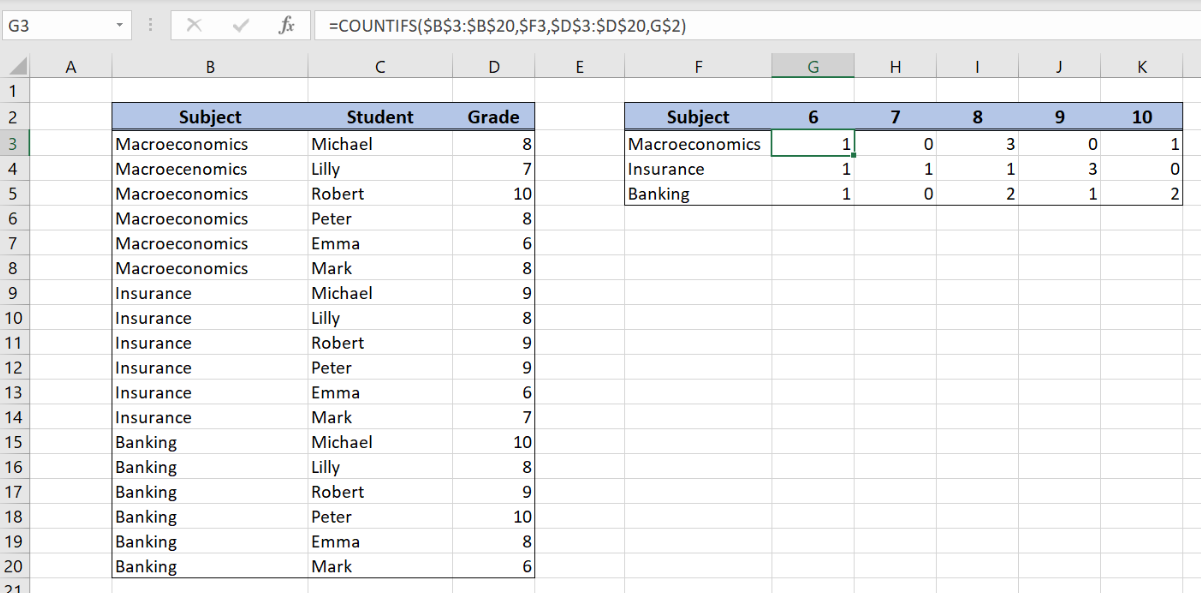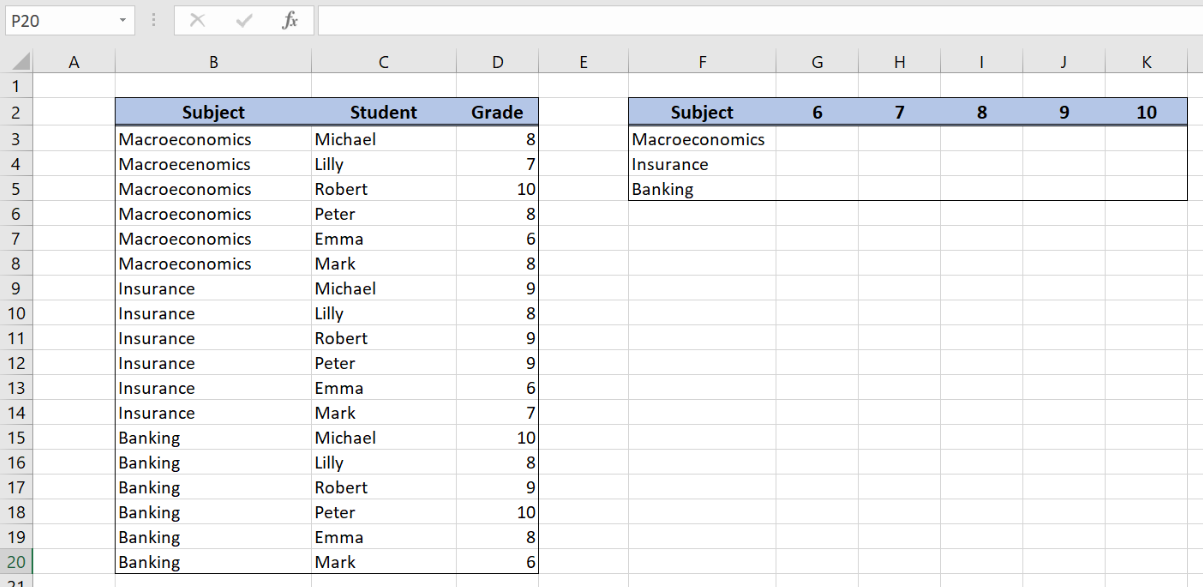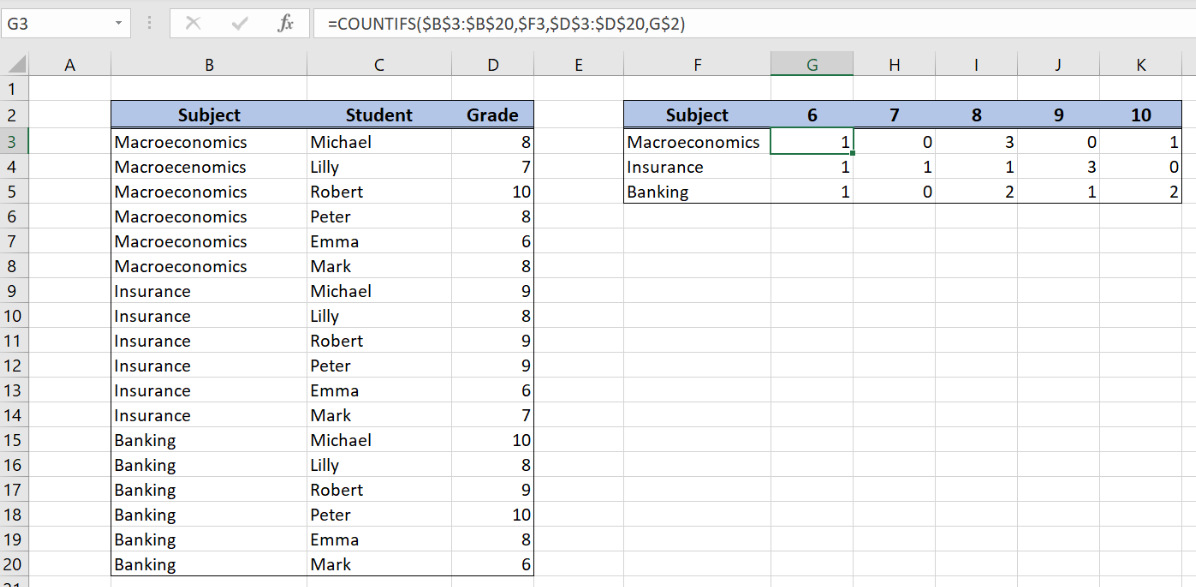Get instant live expert help with Excel or Google Sheets“My Excelchat expert helped me in less than 20 minutes, saving me what would have been 5 hours of work!”

#### Post your problem and you’ll get Expert help in seconds.

Your message must be at least 40 characters
Our professional Expert are available now. Your privacy is guaranteed.

# Two-way summary count with COUNTIFS

Excel allows a user to create two-way summary count by using the COUNTIFS function. This function allows us to count multiple columns. This step by step tutorial will assist all levels of Excel users in creating a two-way summary count.Figure 1. The result of the COUNTIFS function

## Syntax of the COUNTIFS Formula

The generic formula for the COUNTIFS function is:

`=COUNTIFS(criteria_range1, criteria1, criteria_range2, criteria2, ... )`

The parameters of the COUNTIFS function are:

• criteria_range1, criteria_range2 – ranges where we want to apply our criteria
• criteria1, criteria2 – a criteria in criteria ranges which we want to coun

## Setting up Our Data for the COUNTIFS FunctionFigure 2. Data that we will use in the COUNTIFS example

In the data table, we have 3 columns: “Subject” (column B), “Student” (column C) and “Grade” (column D). In the two-way summary table (F2:K5), we want to count grades per subjects.

## Create a two-way summary count with the COUNTIFS table

In our example, we want to count how many different grades (6, 7, 8, 9 and 10)  we have for every subject (Macroeconomics, Insurance and Banking).

The formula looks like:

`=COUNTIFS(\$B\$3:\$B\$20, \$F3, \$D\$3:\$D\$20, G\$2)`

The first criteria is the subject, while the second is the grade. The parameter criteria_range1 is \$B\$3:\$B\$20 and the criteria1 is in the cell F3. The parameter criteria_range2 is \$D\$3:\$D\$20 and the criteria2 is G2.

We must fix both criteria ranges, as they are not changing when we copy the formula across the cells. For criteria1 we must fix the column, as only the row is changing. For criteria2 we must fix the row because only the column is changing.

First, we need to drag the formula right to the other cells in the columns and then all the row down to the other rows.

To apply the COUNTIFS function, we need to follow these steps:

• Select cell G3 and click on it
• Insert the formula: `=COUNTIFS(\$B\$3:\$B\$20, \$F3, \$D\$3:\$D\$20, G\$2)`
• Press enter
• Drag the formula right to the other cells in the row by clicking and dragging the little “+” icon at the bottom-right of the cell to the right
• Select the whole row with the formula (G3:K3). Drag the formula down to the other cells in the columns by clicking and dragging the little “+” icon at the bottom-right of the cell.Figure 3. Creating the two-way summary count with the COUNTIFS table

As a result, we got grades counted for all the subjects in the resulting table.

Most of the time, the problem you will need to solve will be more complex than a simple application of a formula or function. If you want to save hours of research and frustration, try our live Excelchat service! Our Excel Experts are available 24/7 to answer any Excel question you may have. We guarantee a connection within 30 seconds and a customized solution within 20 minutes.

### Did this post not answer your question? Get a solution from connecting with the expert.Another blog reader asked this question today on Excelchat:
Solution examplesi need a formula to find values in column f in worksheet 1 and count them.
Solved by B. A. in 20 minsI have a spreadsheet that contains a list of names/IDs and I want to generate a formula that inserts into a cell the number of times a name or ID appears on that list. Either insert a column into that spreadsheet or transfer that info into a column on a different spreadsheet. Either way would be fine.
Solved by O. L. in 18 minsi need a formula to count values that meet two conditions: one if a value is contained in a cell and another one is cells aren't empty
Solved by X. Q. in 20 minsGreetings, I am trying to find a formula in excel that will return the number of students attending courses. My data is on one sheet and my report will be on another sheet. The report is formated with the students list in a coulmn (far left), the courses are list in a row (top). My data is formatted with all columns, courses in column in "A" and students in column "B". Thanks
Solved by E. C. in 27 minsin range b2:o2, I am trying to write a formula to count if the values are equal to 1 thru 27.
Solved by E. L. in 52 mins## Subscribe to Excelchat.coAnother blog reader asked this question today on Excelchat: